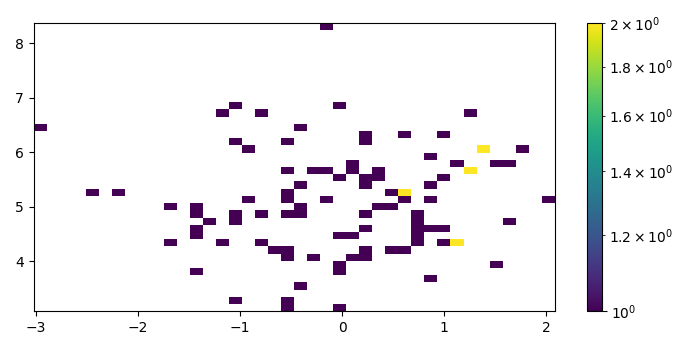# How to add a colorbar for a hist2d plot in Matplotlib?

To add a colorbar for hist2d plot, we can pass a scalar mappable object to colorbar() method's argument.

## Steps

• Create x and y data points using numpy.

• Create a figure and a set of subplots using subplots() method.

• Make a 2D histogram plot using hist2d() method.

• Create a colorbar for a hist2d scalar mappable instance.

• To display the figure, use show() method.

## Example

import numpy as np
from matplotlib import pyplot as plt, colors
plt.rcParams["figure.figsize"] = [7.00, 3.50]
plt.rcParams["figure.autolayout"] = True
x = np.random.randn(100)
y = np.random.randn(100) + 5
fig, ax = plt.subplots()
hh = ax.hist2d(x, y, bins=40, norm=colors.LogNorm())
fig.colorbar(hh, ax=ax)
plt.show()

## Output